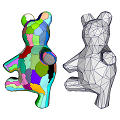CGAL 5.4.4 - Triangulated Surface Mesh Approximation
Triangulated Surface Mesh Approximation ReferencePierre Alliez, David Cohen-Steiner, Lingjie Zhu
This package implements the Variational Shape Approximation method to approximate an input surface triangle mesh by a simpler surface triangle mesh. The algorithm proceeds by iterative clustering of triangles, the clustering process being seeded randomly, incrementally or hierarchically. While the default function runs an automated version of the algorithm, interactive control is possible via a class interface. The API is flexible and can be extended to user-defined proxies and error metrics.
Introduced in: CGAL 4.14
BibTeX: cgal:az-tsma-23a
Depends on: Eigen
Windows Demo: Polyhedron demo
Common Demo Dlls: dlls

## Parameters

Optional parameters of the functions of this package are implemented as BGL named parameters. The page Named Parameters describes their usage.

## Concepts

• ErrorMetricProxy

## Main Functions

• CGAL::Surface_mesh_approximation::approximate_triangle_mesh()

## Classes

• CGAL::Surface_mesh_approximation::L21_metric_plane_proxy
• CGAL::Surface_mesh_approximation::L2_metric_plane_proxy
• CGAL::Variational_shape_approximation

Concepts

## Classes

class  CGAL::Surface_mesh_approximation::L21_metric_plane_proxy< TriangleMesh, VertexPointMap, GeomTraits >
Approximation L21 metric of vector proxy. More...

class  CGAL::Surface_mesh_approximation::L2_metric_plane_proxy< TriangleMesh, VertexPointMap, GeomTraits >
Approximation L2 metric of plane proxy. More...

class  CGAL::Variational_shape_approximation< TriangleMesh, VertexPointMap, ErrorMetricProxy, GeomTraits, Concurrency_tag >
Main class for Variational Shape Approximation algorithm. More...

## Enumerations

enum  CGAL::Surface_mesh_approximation::Verbose_level { CGAL::Surface_mesh_approximation::SILENT, CGAL::Surface_mesh_approximation::MAIN_STEPS, CGAL::Surface_mesh_approximation::VERBOSE }
Verbose level enumeration. More...

enum  CGAL::Surface_mesh_approximation::Seeding_method { CGAL::Surface_mesh_approximation::RANDOM, CGAL::Surface_mesh_approximation::INCREMENTAL, CGAL::Surface_mesh_approximation::HIERARCHICAL }
Seeding method enumeration for Variational Shape Approximation algorithm. More...

## Functions

template<typename TriangleMesh , typename NamedParameters >
bool CGAL::Surface_mesh_approximation::approximate_triangle_mesh (const TriangleMesh &tm, const NamedParameters &np)
approximates the input mesh with plane proxies. More...

## ◆ Seeding_method

#include <CGAL/Variational_shape_approximation.h>

Seeding method enumeration for Variational Shape Approximation algorithm.

Enumerator
RANDOM

Random seeding.

INCREMENTAL

Incremental seeding.

HIERARCHICAL

Hierarchical seeding.

## ◆ Verbose_level

#include <CGAL/Surface_mesh_approximation/approximate_triangle_mesh.h>

Verbose level enumeration.

Enumerator
SILENT

Silent.

MAIN_STEPS

Main steps.

VERBOSE

Verbose.

## ◆ approximate_triangle_mesh()

template<typename TriangleMesh , typename NamedParameters >
 bool CGAL::Surface_mesh_approximation::approximate_triangle_mesh ( const TriangleMesh & tm, const NamedParameters & np )

#include <CGAL/Surface_mesh_approximation/approximate_triangle_mesh.h>

approximates the input mesh with plane proxies.

This function uses the Variational Shape Approximation algorithm described in  to approximate a triangle surface mesh, with indexed triangles as output.

Template Parameters
 TriangleMesh model of FaceListGraph NamedParameters a sequence of Named Parameters
Parameters
 tm triangle surface mesh to be approximated np an optional sequence of Named Parameters among the ones listed below
Returns
true if the indexed triangles represent a 2-manifold, oriented surface mesh, and false otherwise.
Approximation Named Parameters
 an instance of a geometric traits class Type: a model of Kernel Default: a CGAL Kernel deduced from the point type, using CGAL::Kernel_traits Extra: Exact constructions kernels are not supported by this function. a property map associating points to the vertices of tm Type: a class model of ReadablePropertyMap with boost::graph_traits::vertex_descriptor as key type and Point_3 as value type Default: boost::get(CGAL::vertex_point, tm) Extra: If this parameter is omitted, an internal property map for CGAL::vertex_point_t must be available in TriangleMesh. the verbose level Type: CGAL::Surface_mesh_approximation::Verbose_level Default: CGAL::Surface_mesh_approximation::SILENT the selection of seeding method Type: CGAL::Surface_mesh_approximation::Seeding_method Default: CGAL::Surface_mesh_approximation::HIERARCHICAL the maximum number of proxies used to approximate the input mesh Type: std::size_t Default: num_faces(tm) / 3, used when min_error_drop is also not provided the minimum error drop of the approximation, expressed as the ratio between two iterations of proxy addition Type: geom_traits::FT Default: 0.1, used when max_number_of_proxies is also not provided the number of relaxation iterations interleaved within seeding Type: std::size_t Default: 5 the number of partitioning and fitting iterations after seeding Type: std::size_t Default: std::min(std::max(number_of_faces / max_number_of_proxies, 20), 60)
Meshing Named Parameters
 the chord subdivision ratio threshold to the chord length or average edge length Type: geom_traits::FT Default: 5.0 If true, the subdivision_ratio is the ratio of the furthest vertex distance to the chord length, otherwise is the average edge length Type: Boolean Default: false If true, the subdivision_ratio is weighted by dihedral angle Type: Boolean Default: false If true, optimize the anchor locations Type: Boolean Default: true If true, use PCA plane fitting, otherwise use the default area averaged plane parameters Type: Boolean Default: false
Output Named Parameters
 a property map to output the proxy index of each face of the input polygon mesh Type: a model of WritablePropertyMap with boost::graph_traits::face_descriptor as key and std::size_t as value type Default: no output operation is performed Extra: A proxy is a set of connected faces which are placed under the same proxy patch (see Figure 74.3) Extra: The proxy-ids are contiguous in range [0, number_of_proxies - 1] an OutputIterator to put proxies in Type: a class model of OutputIterator with CGAL::Surface_mesh_approximation::L21_metric_vector_proxy_no_area_weighting::Proxy value type Default: no output operation is performed an OutputIterator to put anchor points in Type: a class model of OutputIterator with geom_traits::Point_3 value type Default: no output operation is performed an OutputIterator to put indexed triangles in Type: a class model of OutputIterator with std::array value type Default: no output operation is performed
Examples:
Surface_mesh_approximation/vsa_approximation_2_example.cpp, Surface_mesh_approximation/vsa_approximation_example.cpp, Surface_mesh_approximation/vsa_segmentation_example.cpp, and Surface_mesh_approximation/vsa_simple_approximation_example.cpp.